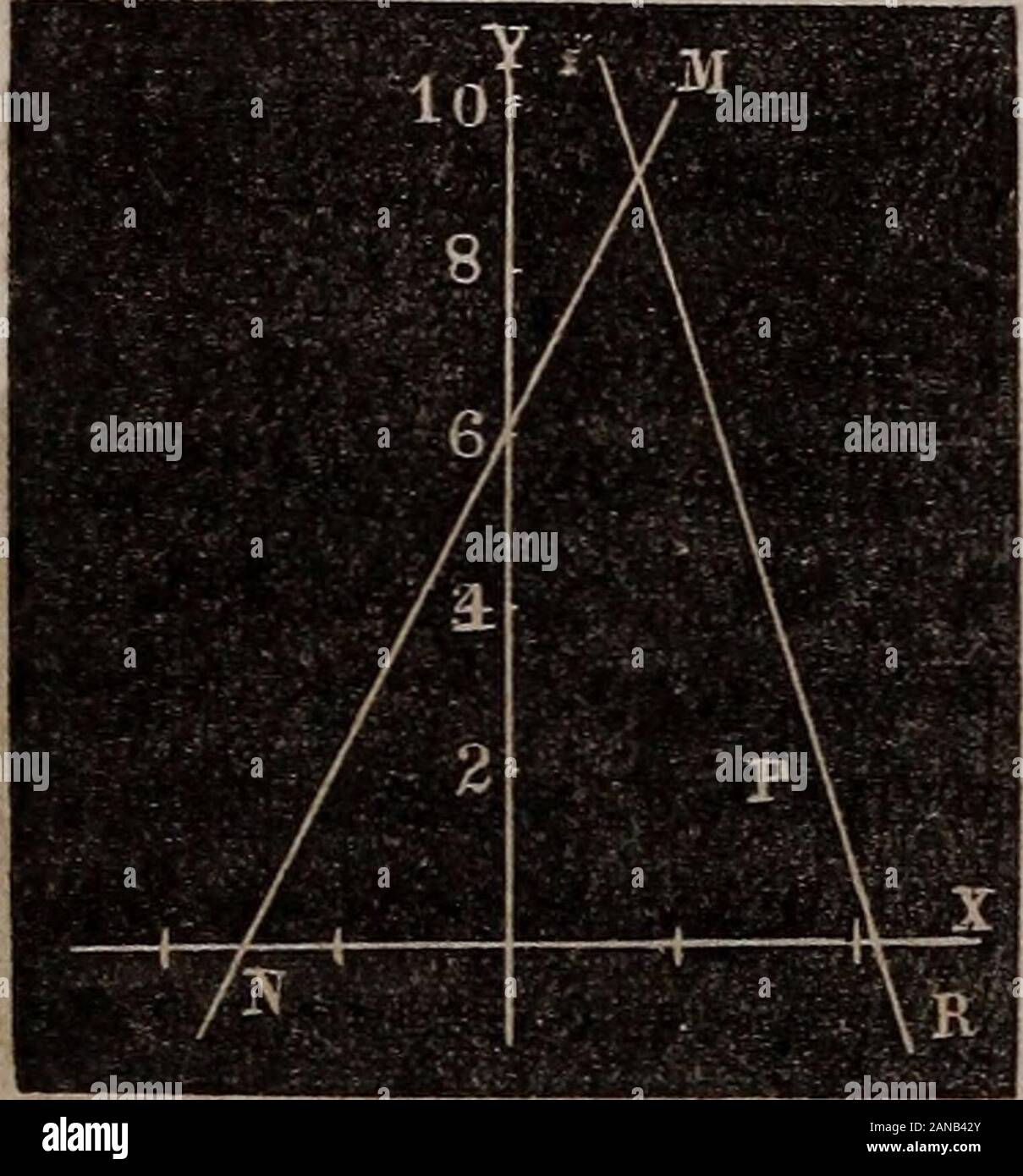# Elements of analytical geometry and the differential and integral calculus . g manner : An infinite quantity cannot be increased, therefore on thata-^m -L m supposition becomes — or -ma —ma Application^.—To make sure that we comprehend this propo-sition and its resulting equation, we give the following example :The equation of a given line is y=2:c-4-6. Draw another line that will in-tersect this at an angle of 45° andpass through a given point P,whose co-ordinates are x=^, 2/=2.Draw the line J/IVcorrespondingto the equation y=^x--6. Locatethe point jP from its given co-or-dinates. Because th## Image details

Contributor:

The Reading Room / Alamy Stock Photo

Image ID:

2ANB42Y

File size:

Releases:

Model - no | Property - noDo I need a release?

Dimensions:

1525 x 1639 px | 25.8 x 27.8 cm | 10.2 x 10.9 inches | 150dpi

This image is a public domain image, which means either that copyright has expired in the image or the copyright holder has waived their copyright. Alamy charges you a fee for access to the high resolution copy of the image.

This image could have imperfections as it’s either historical or reportage.

Elements of analytical geometry and the differential and integral calculus . g manner : An infinite quantity cannot be increased, therefore on thata-^m -L m supposition becomes — or -ma —ma Application^.—To make sure that we comprehend this propo-sition and its resulting equation, we give the following example :The equation of a given line is y=2:c-4-6. Draw another line that will in-tersect this at an angle of 45° andpass through a given point P, whose co-ordinates are x=^, 2/=2.Draw the line J/IVcorrespondingto the equation y=^x--6. Locatethe point jP from its given co-or-dinates. Because the angle of intersectionis to be 45°, m=l, a=2. Substituting these values in (4) we havey~2=~3(x—3^).Or 7/=—3x-{-12i. Constructing the line MB correSj.onding with this equation, we perceive it must pass through F and make the angle iOfi?45°, as was required. The teacher can propose any number of like examples. Corollary 2. Equation (3) shows the tangent of the angleof the inclination of any two lines whose tangents are a and a.That is, we have in o-eneral terms. --aaIn case the two lines are parallel m=0. Whence «=«, anobvious result. STRAIGHT LINES. 19 In case the two lines are perpendicular to each other, m mustbe infinite, and therefore we must put l-f-aa=0 to correspond with this hypothesis, and this gives aa result found in Cor. 1. To show the practical value of this equation we require theangle of inclination of the two lines represented by the equationsyr=^x—6, and y=—x-{-9, . Here a=3 and a=—1. Whence41—3 This is the natural tangent of the angle sought, and if wehave not a table of natural tangents at hand, we will take thelog. of the number and add 10 to the index, then we shall havein the present example 10.301030 for the log. tangent whichcorresponds to 63° 26 6 nearly. The minm sign merely indicates the position of the angle, itis hdow the angular point. PROPOSITIOJ^ VI. To find the co-ordinates which will locate the point of intersec-tion of

Save up to 70% with our image packs